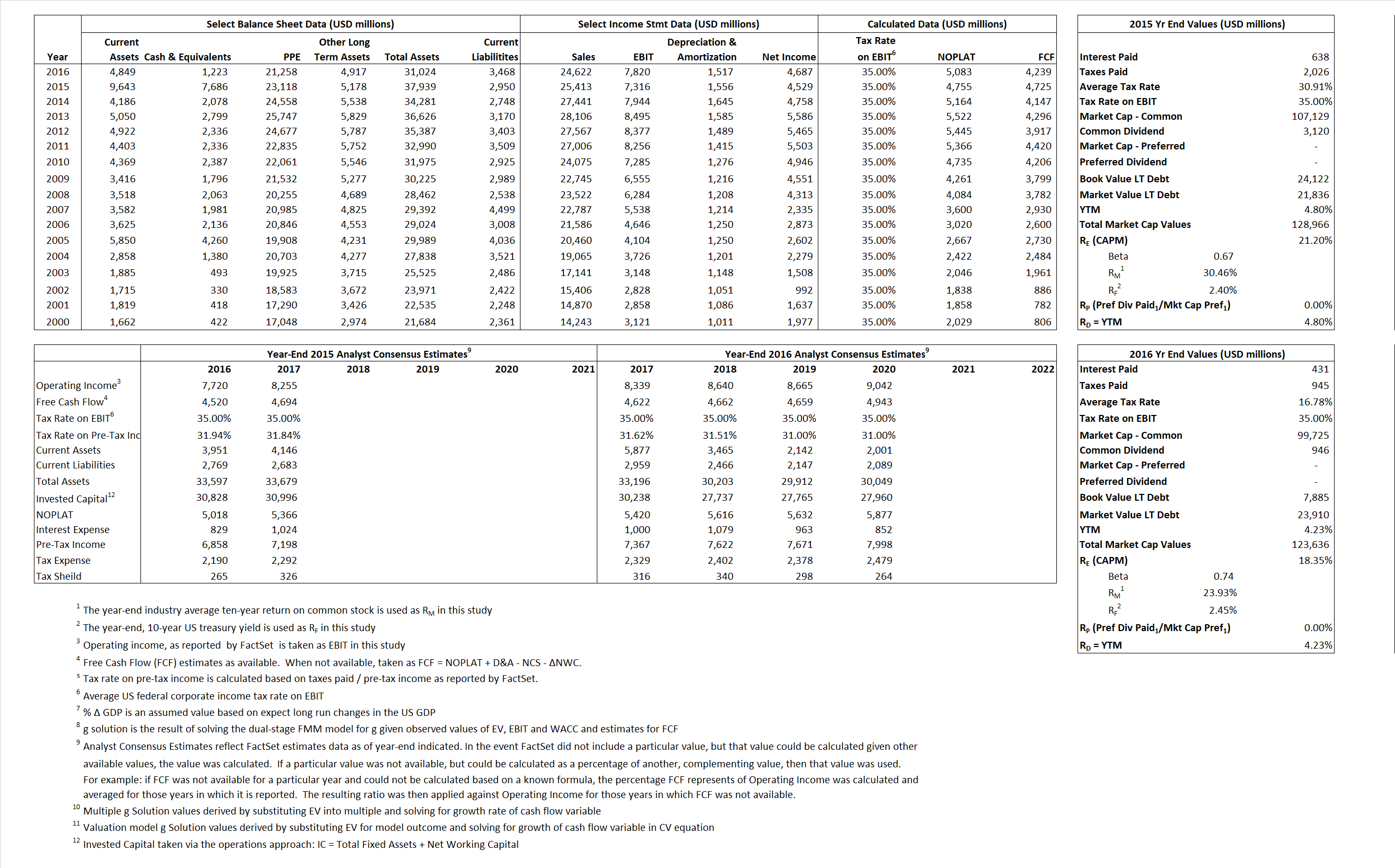# McDonald’s Corporation

## Analyst Listing

The following analysts provide coverage for the subject firm as of May 2016:

 Broker Analyst Analyst Email Longbow Research Alton K. Stump astump@longbowresearch.com Cowen & Company Andrew M. Charles andrew.charles@cowen.com Jefferies Andy Barish abarish@jefferies.com Deutsche Bank Research Brett Levy brett.levy@db.com Oppenheimer Brian Bittner brian.bittner@opco.com Raymond James Brian Vaccaro brian.vaccaro@raymondjames.com RBC Capital Markets David Palmer david.palmer@rbccm.com SunTrust Robinson Humphrey Jake Bartlett jake.bartlett@suntrust.com Credit Suisse Jason West jason.west@credit-suisse.com Wells Fargo Securities Jeff Farmer jeff.farmer@wellsfargo.com CRT Capital Group Lynne Collier lcollier@sterneageecrt.com Nomura Research Mark Kalinowski mark.kalinowski@nomura.com Evercore ISI Matt McGinley matt.mcginley@evercoreisi.com Guggenheim Securities Matthew J. Difrisco matthew.difrisco@guggenheimpartners.com Piper Jaffray Nicole Miller Regan nmr@pjc.com Stifel Nicolaus Paul Westra paul.westra@stifel.com BTIG Peter Saleh psaleh@btig.com Stephens Inc Will Slabaugh will.slabaugh@stephens.com

## Primary Input Data## Derived Input Data

### Equational Form

Net Operating Profit Less Adjusted Taxes NOPLAT 4,755 5,083$NOPLAT\, =\, EBIT\, x\, (1 \,-\, Avg \,\,Tax\,\, Rate\,\, on\,\, EBIT)$
Free Cash Flow FCF 4,725 4,239$FCF\,=NOPLAT\,+\,Non-Cash\,Expenses-\Delta NWC\,-\,NCS$
Tax Shield TS 197 72$TS\,=\,Interest\,\,Paid\,\,x\,\, Avg \,\,Tax\,\,Rate\,\, on\,\, Pre-Tax\,\, Income$
Invested Capital IC 34,988 27,556$IC\,=\,Fixed\,\,Operating\,\,Assets\,\,+\,\,Net\,\, Working\,\, Capital$
Return on Invested Capital ROIC 13.59% 18.45%$ROIC\,=\,\frac { NOPLAT }{ IC }$
Net Investment NetInv 5,011 (5,915)$NetInv\,=\,{ {IC}_{1}}-{{IC}_{0}}+Depreciation$
Investment Rate IR  105.36% -116.38%$IR\,=\,\frac {NetInv}{NOPLAT}$
Weighted Average Cost of Capital
WACCMarket 18.17%  15.48%$WACC\,=\,\frac { E }{ V } { R }_{ E }\,+\,\frac { P }{ V } { R }_{ P }\,+\,\frac { D }{ V } { R }_{ D }\left( 1- Avg\,\, Tax\,\,Rate\,\,on\,\,Pre-Tax\,\,Income \right)$
WACCBook  6.18%    6.27%
Enterprise value
EVMarket  121,280  122,413$EV\,=\,Market\,\,Cap\,\,Equity\,+\,\,Long\,\,Term\,\,Debt\,-\,Cash$
EVBook  130,028   106,387
Long-Run Growth
g = IR x ROIC
14.32%  -21.47% Long-run growth rates of the income variable are used in the Continuing Value portion of the valuation models.
g = %$\Delta$ GDP   2.50%   2.50%
Margin from Operations M  28.79%  31.76%$M\,\,=\,\,\frac{EBIT}{SALES}$
Depreciation/Amortization Rate D  17.54%  16.25%$D\,\,=\,\,\frac{D+A}{EBITDA}$

## Valuation Multiple Outcomes

The outcomes presented in this study are the result of original input data, derived data, and synthesized inputs.

### model g solution

12/31/2015 12/31/2016 12/31/2015 12/31/2016 12/31/2015 12/31/2016

EV/SALES$\frac {EV}{Sales} \,= \,\frac{ROIC\, -\, g}{ROIC\,(WACC\,-\,g)}\,(1\,-\,T)\,(M)$

4.77  4.97  20.03%  14.62%  19.46%  15.05%

EV/EBITDA$\frac {EV}{EBITDA} \,= \,\frac{ROIC\, -\, g}{ROIC\,(WACC\,-\,g)}\,(1\,-\,T)\,(1\,-\,D)$

13.67 13.11 20.03%  14.62% 19.46% 15.05%

EV/NOPLAT$\frac {EV}{NOPLAT} \,= \,\frac{ROIC\, -\, g}{ROIC\,(WACC\,-\,g)}$

25.50 24.08 20.03% 14.62% 19.46% 15.05%

EV/FCFOPS$\frac {EV}{FCF_{OPS}} \,= \,\frac{ROIC\, -\, g}{ROIC\,(WACC\,-\,g)}\,(1\,-\,T)$

25.67 28.88 20.03% 14.62% 19.46% 15.05%

EV/EBIT$\frac {EV}{EBIT} \,= \,\frac{ROIC\, -\, g}{ROIC\,(WACC\,-\,g)}\,(1\,-\,T)$

16.58 15.65 20.03% 14.62% 19.46% 15.05%

EV/IC$\frac {EV}{IC} \,= \,\frac{ROIC\, -\, g}{WACC\,-\,g}$

3.47 4.44 20.03% 14.62% 19.46% 15.05%